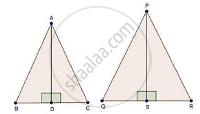Share

Books Shortlist
Your shortlist is empty

# Solution for The Corresponding Altitudes of Two Similar Triangles Are 6 Cm and 9 Cm Respectively. Find the Ratio of Their Areas. - CBSE Class 10 - Mathematics

#### Question

The corresponding altitudes of two similar triangles are 6 cm and 9 cm respectively. Find the ratio of their areas.

#### SolutionWe have,

ΔABC ~ ΔPQR

AD = 6 cm

And, PS = 9 cm

By area of similar triangle theorem

("Area"(triangleABC))/("Area"(trianglePQR))="AB"^2/"PQ"^2              ........(i)

In ΔABD and ΔPQS

∠B = ∠Q [ΔABC ~ ΔPQR]

∠ADB = ∠PSQ [Each 90°]

Then, ΔABD ~ ΔPQS [By AA similarity]

therefore"AB"/"PQ"="AD"/"PS"               [Corresponding parts of similar Δ are proportional]

rArr"AB"/"PQ"=6/9

rArr"AB"/"PQ"=2/3              ......(ii)

Compare equations (i) and (ii)

("Area"(triangleABC))/("Area"(trianglePQR))=(2/3)^2=4/9

Is there an error in this question or solution?

#### Video TutorialsVIEW ALL 

Solution for question: The Corresponding Altitudes of Two Similar Triangles Are 6 Cm and 9 Cm Respectively. Find the Ratio of Their Areas. concept: Areas of Similar Triangles. For the course CBSE
S